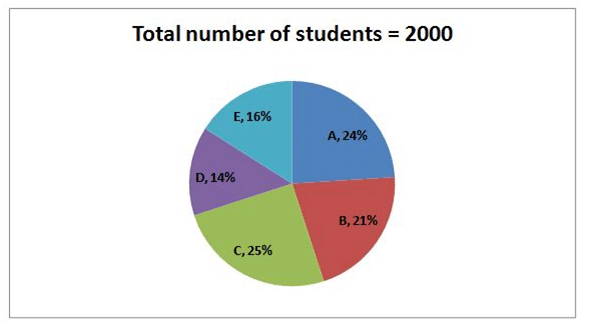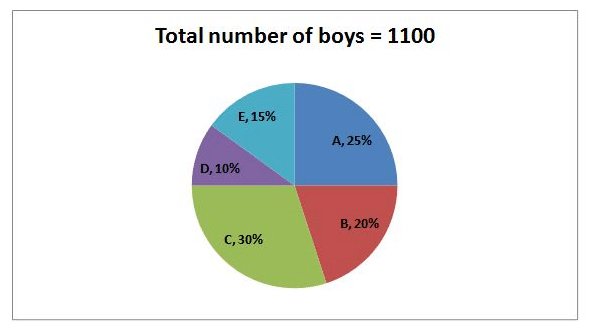# IBPS PO Prelims Quantitative Aptitude (Day-02)

Dear Aspirants, Our IBPS Guide team is providing new series of Quantitative Aptitude Questions for IBPS PO Prelims 2020 so the aspirants can practice it on a daily basis. These questions are framed by our skilled experts after understanding your needs thoroughly. Aspirants can practice these new series questions daily to familiarize with the exact exam pattern and make your preparation effective.

Start Quiz

Data Interpretation

Direction (1-5): Study the following information carefully and answer the questions given below.

The first pie chart shows the total number of students in five different colleges and the second pie chart shows the number of boys in different colleges.1) What is the difference between the number of girls in A and E?

A) 20

B) 40

C) 50

D) 60

E) None of these

2) Which of the following colleges has the same number of girl students?

A) A and C

B) C and D

C) E and A

D) B and E

E) None of these

3) The number of boys in D is approximately what percent of the total number of students in B?

A) 24%

B) 26%

C) 28%

D) 30%

E) 32%

4) What is the difference between the average number of boys and girls in all the colleges together?

A) 30

B) 20

C) 50

D) 60

E) None of these

5) The number of boys from B is approximately what percent of the number of boys in C?

A) 33.33%

B) 44.44%

C) 55.56%

D) 66.67%

E) 77.78%

Quantity based

Direction (6-7): Following questions have two quantities as Quantity I and Quantity II. You have to determine the relationship between them and give answer as,

6)

Quantity I: The marked price of the shirt is Rs.6300 and the shopkeeper allows the discount 10% while gets the profit 8%. The cost price of the shirt is what percent of the marked price of the shirt?

Quantity II: The marked price and selling price of the laptop is Rs.12000 and Rs.1920 respectively. If the shopkeeper offers two successive discounts x% and 20% respectively due to some damages in the laptop, then find the value of x?

A) Quantity I > Quantity II

B) Quantity I ≥ Quantity II

C) Quantity I = Quantity II

D) Quantity I < Quantity II

E) Quantity I ≤ Quantity II

7)

Quantity I: If the ratio of the length and breadth of the rectangle is 2: 1 and the perimeter of the rectangle is 42 cm. If the breadth of the rectangle is equal to the radius of the circle, then what is the circumference of the circle?

Quantity II: If the difference between the radius of the circle and side of the square is 4 cm and the area of the circle is 154 cm, then what is the perimeter of the square if the side of the square is greater than radius of the circle?

A) Quantity I > Quantity II

B) Quantity I ≥ Quantity II

C) Quantity I = Quantity II

D) Quantity I < Quantity II

E) Quantity I ≤ Quantity II

Data Sufficiency

Direction (8-10): Following questions contain two statements as statement I and statement II. You have to determine which statement/s is/are necessary to answer the question and give answer as,

8) What is the length of the train?

Statement I: The speed of the train is 60 kmph and the train crosses a man running opposite direction at the speed of 20 kmph in 18 seconds.

Statement II: The train crosses the platform in 36 seconds and the length of the platform is 500m.

A) The data in statement I alone is sufficient to answer the question, while the data in statement II alone is not sufficient to answer the question

B) The data in statement II alone is sufficient to answer the question, while the data in statement I alone is not sufficient to answer the question

C) The data either in statement I alone or in statement II alone is sufficient to answer the question

D) The data given in both statements I and II together are not sufficient to answer the question

E) The data given in both statements I and II together are necessary to answer the question.

9) What is the number of female employees in the company?

Statement I: The total number of male employees in the company is 1000.

Statement II: The number of male and female employees decreased by 10% and 20% respectively, then the ratio of the male to female employees becomes is 5: 4.

A) The data in statement I alone is sufficient to answer the question, while the data in statement II alone is not sufficient to answer the question

B) The data in statement II alone is sufficient to answer the question, while the data in statement I alone is not sufficient to answer the question

C) The data either in statement I alone or in statement II alone is sufficient to answer the question

D) The data given in both statements I and II together are not sufficient to answer the question

E) The data given in both statements I and II together are necessary to answer the question.

10) Find the two digit positive number?

Statement I: Sum of the digits of the number is 10.

Statement II: Product of the digits is 16.

A) The data in statement I alone is sufficient to answer the question, while the data in statement II alone is not sufficient to answer the question

B) The data in statement II alone is sufficient to answer the question, while the data in statement I alone is not sufficient to answer the question

C) The data either in statement I alone or in statement II alone is sufficient to answer the question

D) The data given in both statements I and II together are not sufficient to answer the question

E) The data given in both statements I and II together are necessary to answer the question.

Direction (1-5):Required difference = 205 – 155 = 50

Required percentage = 110/420 * 100 = 26%

Average number of boys = 1100/5 = 220

Average number of girls = (2000 – 1100)/5 = 900/5 = 180

Difference = 220 – 180 = 40

Required percentage = 220/330 * 100 = 66.67%

Directions (6-7) :

From quantity I,

MP = 6300

6300 * 90/100 = CP * 108/100

CP = 5250

Required percentage = 5250/6300 * 100 = 83%

From quantity II,

MP = 12000

SP = 1920

12000 * (100 – x)/100 * 80/100 = 1920

(100 – x) = 20

x = 80%

Quantity I > quantity II

From quantity I,

Perimeter of the rectangle = 2 * (l + b)

2 * (2x + x) = 42

3x = 21

x = 7 cm

Radius of the circle = 7 cm

Circumference of the circle = 22/7 * 2 * 7 = 44 cm

From quantity II,

Area of the circle = 22/7 * r * r = 154

Radius of the circle = 7 cm

Side of the square = 7 + 4 = 11 cm

Perimeter of the square = 4 * a = 4 * 11 = 44 cm

Quantity I = Quantity II

Directions (8-10) :

From statement I,

X= (60 + 20) * 5/18 * 18 = 400 m

So, Statement I alone is sufficient to the answer the question.

From statement II,

Speed of the train is not given.

So, Statement II alone is not sufficient to the answer the question.

From statement I,

Total number of male = 1000

So, Statement I alone is not sufficient to the answer the question.

From statement II,

The number of male and female employees decreased by 10% and 20% respectively, then the ratio of the male to female employees becomes is 5:4.

So, Statement II alone is not sufficient to the answer the question.

From I and II,

(1000 * 90/100)/x * 80/100 = 5/4

900/x * 80/100 = 5/4

3600 = 400x/100

x = 900

Both statements are necessary to answer the question.

From statement I,

10x + y = 10

So, Statement I alone isnot sufficient to the answer the question

From statement II,

10x * y = 16

So, Statement II alone is not sufficient to the answer the question.